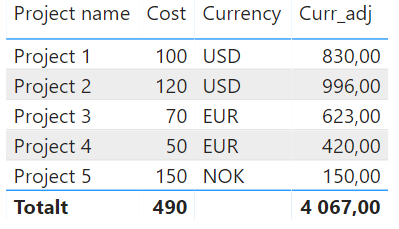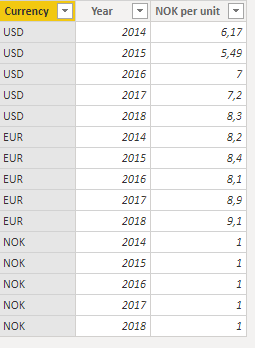cancel
Showing results for
Did you mean:

Fabric is Generally Available. Browse Fabric Presentations. Work towards your Fabric certification with the Cloud Skills Challenge.Helper I

## Sumtotal Currency Conversion Problem

Hi, I am doing a currency conversion with a double lookup that matches on both currency and time. This works fine for the individual rows in the table, however, the sumtotal is wrong. I found that one has to treat sumtotals separately, and that HASONEVALUE() is a good function to ensure this.

The problem is what DAX formula to use for the sumtotal, marked as "?" in the formula below. I have tried several different methods using SUMX(), but I haven't been able to solve it.

Also, I have scoured the web for tutorials and solutions but all currency conversion tutorials seem to have the same issue.

Help would be greatly appreciated!

Note that there is also a filtering here between currency selection "NOK" and "Local Currency".

[Currency selection]="NOK";IF(HASONEVALUE('CapEX Documents'[Budget]);SUM('CapEX Documents'[Budget])*LOOKUPVALUE('Currencies'[NOK per unit];'Currencies'[Currency];MAX('CapEX Documents'[Currency]);'Currencies'[Year];YEAR(MAX('CapEX Documents'[Scheduled start])));"?");
[Currency selection]="Local Currency"; SUM('CapEX Documents'[Budget]))
1 ACCEPTED SOLUTIONHelper I

Solved it!

SUM('Project list'[Cost])*LOOKUPVALUE(Currencies[NOK per unit];Currencies[Currency];MAX('Project list'[Currency]);Currencies[Year];YEAR(MAX('Project list'[Start date])));
SUMX('Project list';CALCULATE(SUM('Project list'[Cost])*LOOKUPVALUE(Currencies[NOK per unit];Currencies[Currency];MAX('Project list'[Currency]);Currencies[Year];YEAR(MAX('Project list'[Start date]))))))

The solution was a combination of SUMX and CALCULATE.
5 REPLIES 5Helper I

Solved it!

SUM('Project list'[Cost])*LOOKUPVALUE(Currencies[NOK per unit];Currencies[Currency];MAX('Project list'[Currency]);Currencies[Year];YEAR(MAX('Project list'[Start date])));
SUMX('Project list';CALCULATE(SUM('Project list'[Cost])*LOOKUPVALUE(Currencies[NOK per unit];Currencies[Currency];MAX('Project list'[Currency]);Currencies[Year];YEAR(MAX('Project list'[Start date]))))))

The solution was a combination of SUMX and CALCULATE.Super User

Hi @oslosa

I don't quite get it.

Is Curr_adj_value a measure?  How exactly are you using it?

Can you clarify this?

This works fine for the individual rows in the table, however, the sumtotal is wrong.

Is table a table visual? Is  sumtotal the total in the visual for the measure? What's in each indivdual row?

Could you share the pbix?

An example would help

Please mark the question solved when done and consider giving kudos if posts are helpful.

CheersHelper I

Hi, yes, Curr_adj_value is a measure. It is connected to two datasets, one is a list wil projects and their cost in local currency, the other is a list of exchange rates for different currencies at different points in time.

The measure is supposed to return the project cost in Local currency when the local currency box is checked and in NOK when the NOK box is checked. When creating a visual, a table, with project names and cost, the measure works perfectly for each individual project in the list, each row. However, the sum total, i.e. the bottom row, returns a bogus number after the conversion to NOK.

The DAX code has to treat this bottom row separately in order for it to work, i.e. that the sumtotal matches the sum of the individual rows/projects, but I need help in writing this part of the DAX code.Super User

Hi @oslosa

It would certainly help if you showed your tables. I'll give you a generic solution, since I get the idea behind the question but I'm not clear on the details:

```Measure =
SUMX ( SUMMARIZE ( Table1, Table1[Col1], Table1[Col2] ), [Your calcs per row] )```

What we're doing here is recreate in the measure what you have in the  table visual. You need all the columns you use in the visual in the summarize. At the total, the summarize will generate a table with all the rows in your visual, and then the sumx will do the calcs per row and sum them all up. At each individual row, the summarize will have only the elements in that row (filter context active). Watch out for context transition when doing the calcs per row.

Please mark the question solved when done and consider giving kudos if posts are helpful.

CheersHelper I

Hi, I have created a dummy PBI that is shareable, but I dont see an upload file button here.

Anyways, here are the visual and two tables the visual gets the data from. The Curr_adj column calculates using the DAX formula below. As you see, the sum total doesnt match the sum of the individual rows in the currency converted column.Curr_adj = SUM('Project list'[Cost])*LOOKUPVALUE(Currencies[NOK per unit];Currencies[Currency];MAX('Project list'[Currency]);Currencies[Year];YEAR(MAX('Project list'[Start date])))Announcements#### Power BI Monthly Update - November 2023

Check out the November 2023 Power BI update to learn about new features.#### Fabric Community News unified experience

Read the latest Fabric Community announcements, including updates on Power BI, Synapse, Data Factory and Data Activator.#### The largest Power BI and Fabric virtual conference

130+ sessions, 130+ speakers, Product managers, MVPs, and experts. All about Power BI and Fabric. Attend online or watch the recordings.Top Solution Authors
Top Kudoed Authors
Users online (2,867)